Related Articles

# Reference Variable in Java

• Difficulty Level : Easy
• Last Updated : 29 May, 2021

Before We Started with the Reference variable we should know about the following facts.

1. When we create an object (instance) of class then space is reserved in heap memory. Let’s understand with the help of an example.

Demo D1 = new Demo();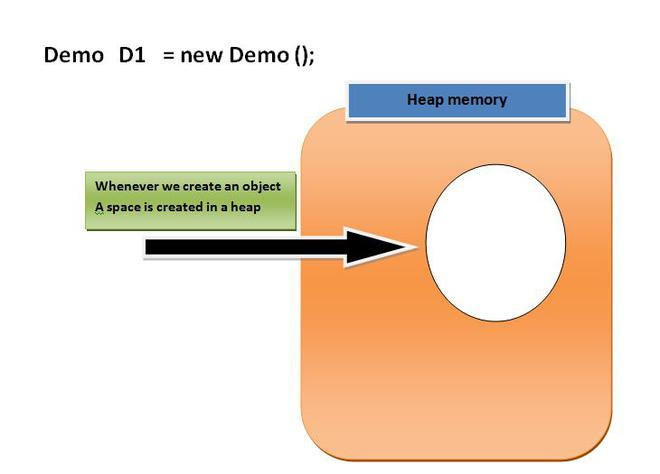Now, The space in the heap Memory is created but the question is how to access that space?.

Then, We create a Pointing element or simply called Reference variable which simply points out the Object(the created space in a Heap Memory).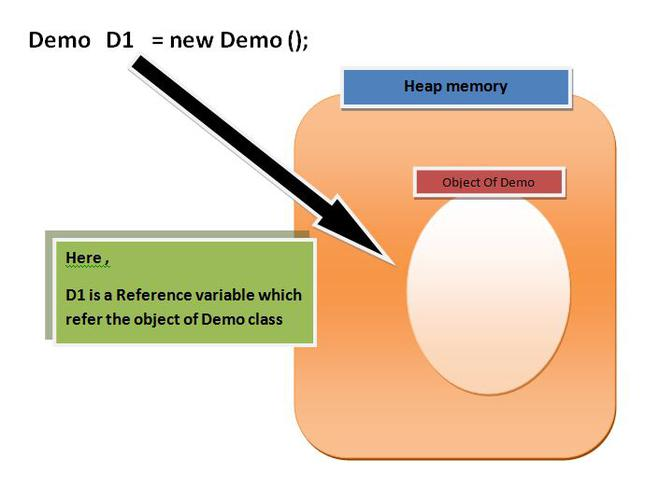Understanding Reference variable

1. Reference variable is used to point object/values.

2. Classes, interfaces, arrays, enumerations, and, annotations are reference types in Java. Reference variables hold the objects/values of reference types in Java.

3. Reference variable can also store null value. By default, if no object is passed to a reference variable then it will store a null value.

4. You can access object members using a reference variable using dot syntax.

<reference variable name >.<instance  variable_name / method_name>

Example:

## Java

 `// Java program to demonstrate reference``// variable in java` `import` `java.io.*;` `class` `Demo {``    ``int` `x = ``10``;``    ``int` `display()``    ``{``        ``System.out.println(``"x = "` `+ x);``        ``return` `0``;``    ``}``}` `class` `Main {``    ``public` `static` `void` `main(String[] args)``    ``{``        ``Demo D1 = ``new` `Demo(); ``// point 1` `        ``System.out.println(D1); ``// point 2` `        ``System.out.println(D1.display()); ``// point 3``    ``}``}`

Output
```Demo@214c265e
x = 10
0```

Let us see what is actually happening step by step.

1. When we create an object of demo class new DEMO();, the default constructor is called and returns a reference of the object, and simply this reference will be stored to the reference variable D1 (As we know that associativity is Right-hand side to left-hand side).

2. The value of a reference variable is a reference. When we attempt to print the value of a reference variable, the output contains the type of the variable and the hash code created for it by Java: the string Demo@214c265e tells us that the given variable is of type Name and its hexadecimal format of hash code is 214c265e.

3. At this point we will access the methods display() of the class demo using our custom reference variable that we created.

BINDING UP : The constructor call returns a value that is a reference to the newly-created object. The equality sign tells the program that the value of the right-hand side expression is to be copied as the value of the variable on the left-hand side. The reference to the newly-created object, returned by the constructor call, is copied as the value of the variable.

## Java

 `import` `java.io.*;``class` `Demo {``    ``int` `x = ``10``;` `    ``int` `display()``    ``{``        ``System.out.println(``"x = "` `+ x);``        ``return` `0``;``    ``}``}` `class` `Main {``    ``public` `static` `void` `main(String[] args)``    ``{``          ``// create instance``        ``Demo D1 = ``new` `Demo();` `        ``// accessing instance(object) variable``        ``System.out.println(D1.x);` `        ``// point 3``        ``// accessing instance(object) method``        ``D1.display();``    ``}``}`

Output
```10
x = 10```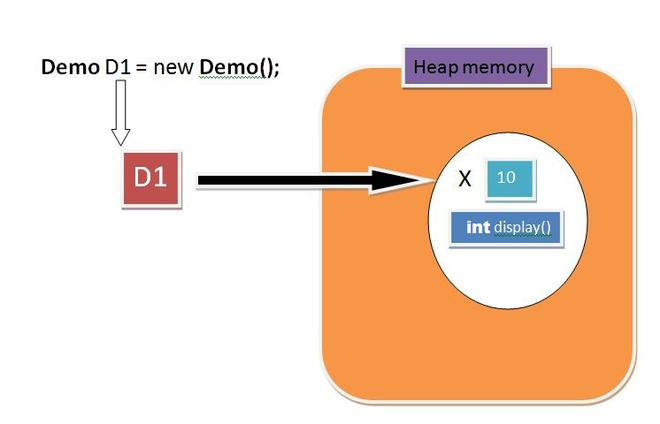## Java

 `// Accessing instance methods` `import` `java.io.*;``class` `Demo {``  ` `    ``int` `x = ``10``;``    ``int` `display()``    ``{``        ``System.out.println(``"x = "` `+ x);``        ``return` `0``;``    ``}``}``class` `Main {``    ``public` `static` `void` `main(String[] args)``    ``{``          ``// create instances``        ``Demo D1 = ``new` `Demo();` `        ``Demo M1 = ``new` `Demo();` `        ``Demo Q1 = ``new` `Demo();``    ``}``}`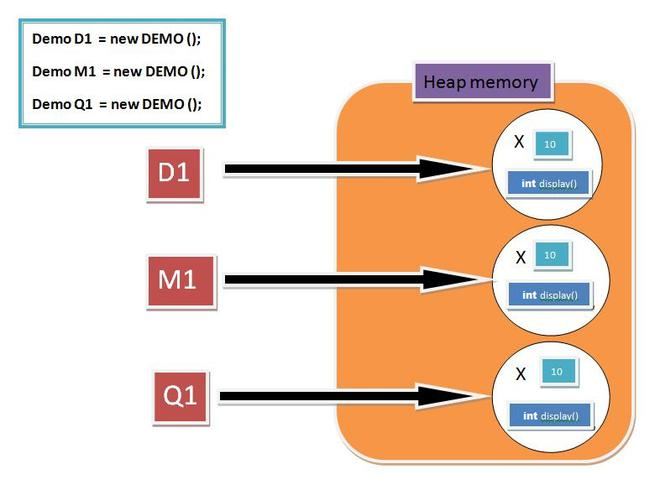## Java

 `// Pointing to same instance memory` `import` `java.io.*;``class` `Demo {``    ``int` `x = ``10``;``    ``int` `display()``    ``{``        ``System.out.println(``"x = "` `+ x);``        ``return` `0``;``    ``}``}``class` `Main {``    ``public` `static` `void` `main(String[] args)``    ``{``        ``// create instance``        ``Demo D1 = ``new` `Demo();` `        ``// point to same reference``        ``Demo G1 = D1;` `        ``Demo M1 = ``new` `Demo();` `        ``Demo Q1 = M1;` `        ``// updating the value of x using G!``        ``// reference variable``        ``G1.x = ``25``;` `        ``System.out.println(G1.x); ``// Point 1` `        ``System.out.println(D1.x); ``// Point 2``    ``}``}`

Output
```25
25```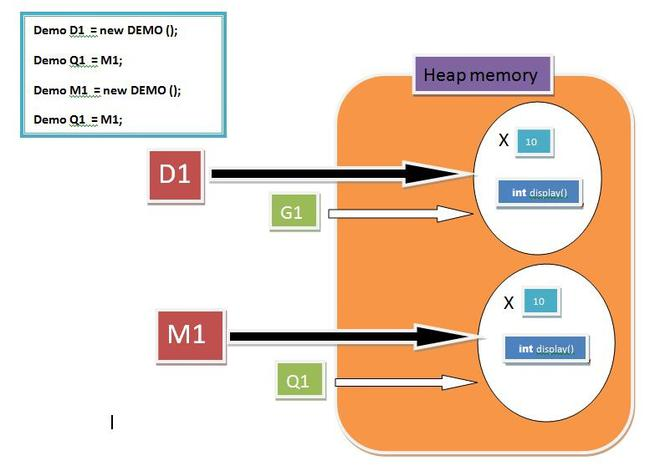Note:

Here we pass G1 and Q1 reference variable point out the same object respectively.<br> Secondly **At Point 1** we try to get the value of the object with G1 reference variable which shows it as **25** and **At Point 2**we try to get the value of an object with D1 reference variable which shows it as **25** as well “” This will prove that the modification in the object can be done by using any reference variable but the condition is it should hold the same reference.

### More on Reference Variable

1. Reference Variable as Method Parameters:

As the value of a primitive variable is directly stored in the variable, whereas the value of a reference variable holds a reference to an object. We also mentioned that assigning a value with the equality sign copies the value (possibly of some variable) on the right-hand side and stores it as the value of the left-hand-side variable. A similar kind of copying occurs during a method call. Regardless of whether the variable is primitive or reference type, a copy of value passed to the method’s argument and copied to that argument.

Note: Java only supports pass by value.

But we know that the reference variable holds the reference of an instance(OBJECT) so a copy of reference passed to the method’s argument.

Example:

## Java

 `// Pass by reference and value` `import` `java.io.*;``class` `Demo {``    ``int` `x = ``10``;``    ``int` `y = ``20``;` `    ``int` `display(Demo A, Demo B)``    ``{``        ``//  Updating value using argument``        ``A.x = ``95``;` `        ``System.out.println(``"x = "` `+ x);` `        ``System.out.println(``"y = "` `+ y);` `        ``return` `0``;``    ``}``}``class` `Main {``    ``public` `static` `void` `main(String[] args)``    ``{``        ``Demo C = ``new` `Demo();` `        ``Demo D = ``new` `Demo();` `        ``// updating value using primary reference``        ``// variable``        ``D.y = ``55``;` `        ``C.display(C, D); ``// POINT 1` `        ``D.display(C, D); ``// POINT 2``    ``}``}`

Output
```x = 95
y = 20
x = 10
y = 55```

SCENE 1 :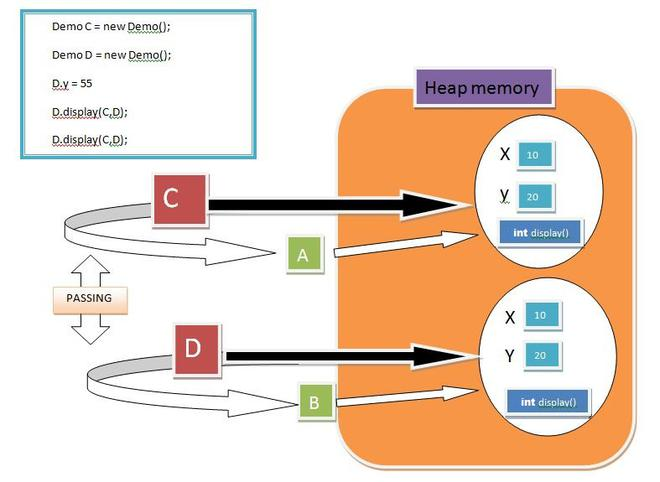SCENE 2: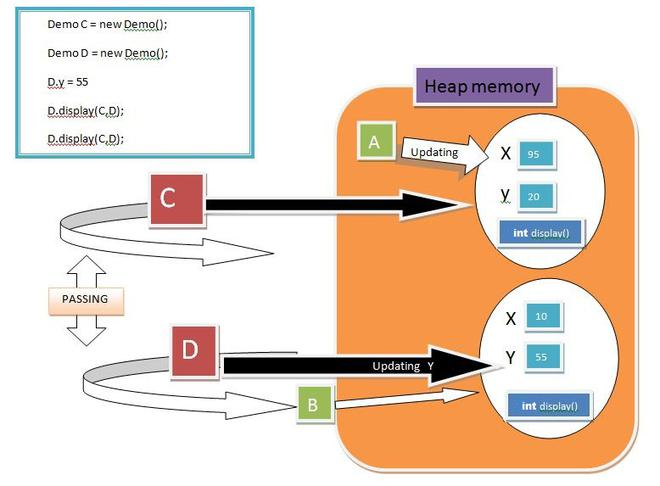Now, What is going on here, when we pass the reference to the method it will copy to the reference variable defined in the method signature and After that, they also have access to the object members. Here, We defined two instances named C and D. Afterwards we pass C and D to the method which further gives reference to A and B

At Point 1: A will update the value of x from 10 to 95, hence C.display() will show 95 20 but in another object D we update the value of x through D only from y =20 to 55, hence D, display() will show 10 and 55.

Note: Any Object Updation will not affect the other object’s member.

2. What if we swap the reference variables with the help of the Swap Method?

The fact is if we try to swap the reference variable, then they just swap their Pointing element there is no effect on the address of reference variable and object(Instance) Space. Let’s Understand It with the help of an example:

## Java

 `// Swapping object references` `import` `java.io.*;``class` `Demo {` `    ``// Swapping Method``    ``int` `Swap(Demo A, Demo B)``    ``{``        ``Demo temp = A;``        ``A = B;``        ``B = temp;``        ``return` `0``;``    ``}``}``class` `Main {``    ``public` `static` `void` `main(String[] args)``    ``{``        ``Demo C = ``new` `Demo();` `        ``Demo D = ``new` `Demo();` `        ``// Passing C and reference variables``        ``// to Swap method``        ``C.Swap(C, D);``    ``}``}`

Here we created, two instances of demo class and passes it to swap method, further those C and D will copy their references to A and B respectively.Before swapping A point to C’s(Object) and B point to D’s(Object). After we perform swapping on A and B, A will now point D’s(Object) and B will Point C’s Object. As described in the figure.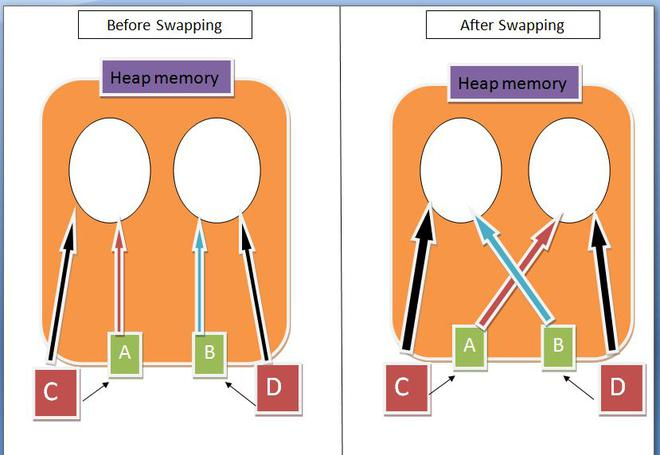Note: There is no swapping between Variables, They only change their References.

3. What if we pass arrays to the method will it be able to update the Actual Array’s values, even we know that a copy of the array is a pass to the formal Array?

The answer is YES, the values will be updated by Formal parameter, The Fact is, When we create an Array, a memory is assigned to the array of the desired size, and it returns the reference of the first array’s element that is the base address that will store to the Formal Array(Method argument). As we learned earlier every pointing reference variable can change or update the object.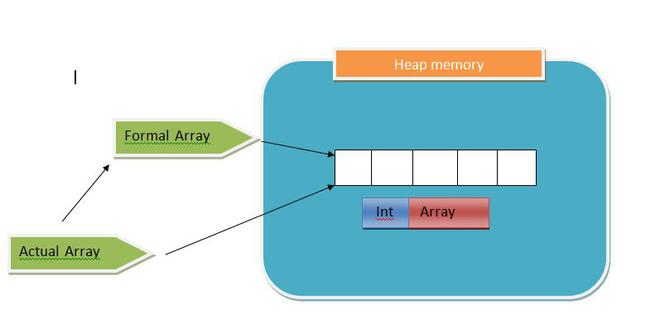Example:

## Java

 `import` `java.io.*;``class` `Demo {``    ``int` `arrayUpdate(``int``[] formalArray)``    ``{``        ``formalArray[``2``] = ``99``;``        ``formalArray[``4``] = ``77``;``        ``return` `0``;``    ``}``}``class` `Main {``    ``public` `static` `void` `main(String[] args)``    ``{``        ``Demo d1 = ``new` `Demo();``        ``int``[] actualArray = { ``1``, ``2``, ``3``, ``4``, ``5` `};` `        ``for` `(``int` `items : actualArray)``            ``System.out.print(items``                             ``+ ``" , "``); ``// printing array` `        ``System.out.println();``        ``d1.arrayUpdate(actualArray);``        ``System.out.println();` `        ``for` `(``int` `items : actualArray)``            ``System.out.print(items``                             ``+ ``" , "``); ``// printing array``    ``}``}`

Output
```1 , 2 , 3 , 4 , 5 ,

1 , 2 , 99 , 4 , 77 , ```

4. this and super keywords are also Pointing Elements.

this keyword. In java, this is a reference variable that refers to the current object.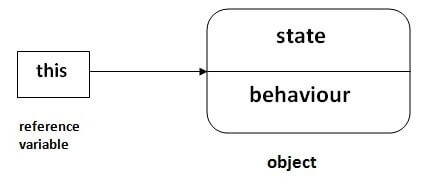super is used to refer immediate parent class instance variable. We can use the super keyword to access the data member or field of the parent class. It is used if parent class and child class have the same fields.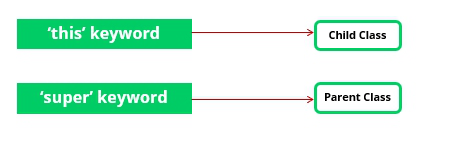5. null value of a reference variable.

Demo obj = null ;

A. The null reference can be set as the value of any reference type variable.

B. The object whose name is obj is referred to by nobody. In other words, the object has become garbage. In the Java programming language, the programmer need not worry about the program’s memory use. From time to time, the automatic garbage collector of the Java language cleans up the objects that have become garbage. If the garbage collection did not happen, the garbage objects would reserve a memory location until the end of the program execution.

## Java

 `// null in java` `import` `java.io.*;``class` `Demo {``    ``int` `x = ``10``;``    ``int` `display()``    ``{``        ``System.out.println(``"x = "` `+ x);``        ``return` `0``;``    ``}``}``class` `Main {``    ``public` `static` `void` `main(String[] args)``    ``{``        ``Demo obj = ``null``;` `        ``// accessing instance(object) method``        ``Kuchbhi.display();``    ``}``}`

Output

```Exception in thread "main" java.lang.NullPointerException
at Main.main(File.java:17)
Java Result: 1```

Here, we try to access objects’ members by a reference variable which is pointing nothing(null), and hence it shows NullPointerException. Now if you get the error, the first step is to look for variables whose value could be null. Fortunately, the error message is useful: it tells which row caused the error. Just try it out yourself!

Attention reader! Don’t stop learning now. Get hold of all the important Java Foundation and Collections concepts with the Fundamentals of Java and Java Collections Course at a student-friendly price and become industry ready. To complete your preparation from learning a language to DS Algo and many more,  please refer Complete Interview Preparation Course.

My Personal Notes arrow_drop_up